Next: Relativistic Electron Theory Up: Scattering Theory Previous: Scattering of Identical Particles

# Exercises

1. Justify Equation (10.5).

2. Justify Equation (10.7).

3. Consider the Green's function for the Helmholtz equation: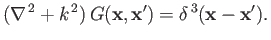1. Assuming, as seems reasonable, that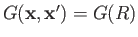, where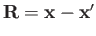, demonstrate that the two independent solutions in the region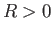are2. Show that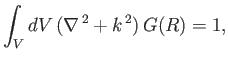whereis a sphere of vanishing radius centered on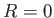. Hence, deduce that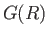is the required Green's function.

1. Demonstrate that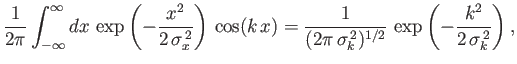where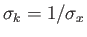.

2. Show that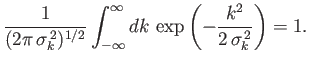3. Finally, by taking the limit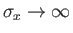, conclude that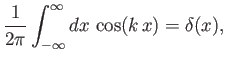where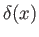is a Dirac delta function.

4. Demonstrate that the probability current associated with the spherical waves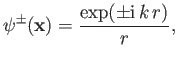where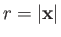, is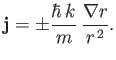Hence, deduce that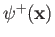and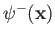correspond to outgoing and ingoing spherical waves, respectively.

5. Justify Equation (10.37).

6. Show that, in the Born approximation, the total scattering cross-section associated with the Yukawa potential, (10.35), is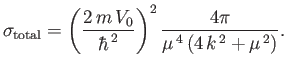7. Consider a scattering potential of the form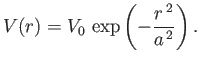Demonstrate, using the Born approximation, that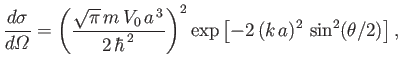and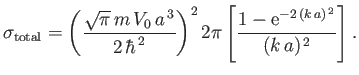8. Show that the differential cross-section for the elastic scattering of a fast electron by the ground state of a hydrogen atom is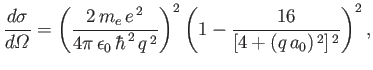where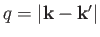, and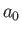is the Bohr radius.

9. Justify Equations (10.57) and (10.59).

10. Demonstrate that, for a spherically symmetric scattering potential,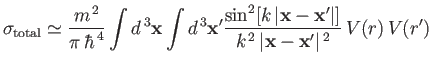in each of the following ways:
1. By directly integrating the differential scattering cross-section obtained from the Born approximation.
2. By utilizing the optical theorem in combination with the first two terms in the Born expansion. [Note that the first term in this expansion is real, and, therefore, does not contribute to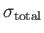.]

11. Justify Equations (10.98) and (10.100).

12. Consider a scattering potential that takes the constant value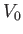for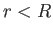, and is zero for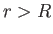, wheremay be either positive or negative. Using the method of partial waves, show that for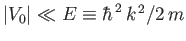, and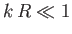,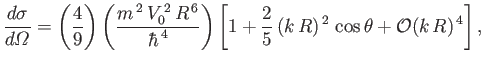and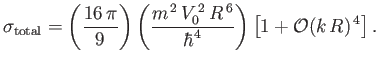13. Consider scattering of particles of mass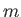and incident wavenumber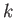by a repulsive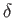-shell potential: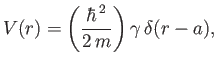where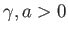. Show that the S-wave phase-shift is given by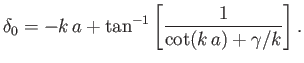Assuming that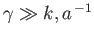, demonstrate that if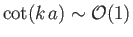then the solution of the previous equation takes the form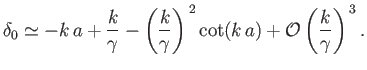Of course, in the limit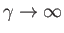, the preceding equation yields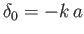, which is the same result obtained when particles are scattered by a hard sphere of radius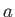. (See Section 10.8.) This is not surprising, because a strong repulsive-shell potential is indistinguishable from hard sphere as far as external particles are concerned.

The previous solution breaks down when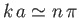, where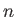is a positive integer. Suppose that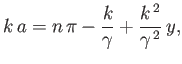where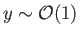. Demonstrate that the S-wave contribution to the total scattering cross-section takes the form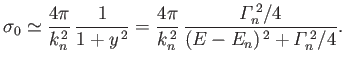where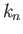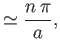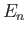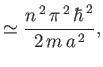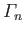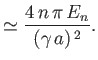Hence, deduce that the net S-wave contribution to the total scattering cross-section is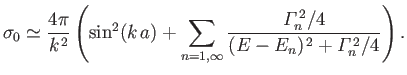Obviously, there are resonant contributions to the cross-section whenever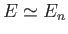. Note that the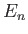are the possible energies of particles trapped within the-shell potential. Hence, the resonances are clearly associated with incident particles tunneling though the-shell and forming transient trapped states. However, the width of the resonances (in energy) decreases strongly as the strength,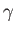, of the shell increases.

14. Consider the mutual scattering of two, counter-propagating, unpolarized, proton beams in the center of mass frame. Making use of the Born approximation, demonstrate that the differential scattering cross-section is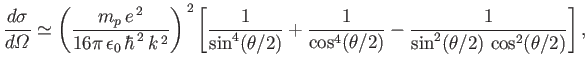where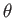is the scattering angle,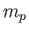the proton mass, and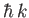the magnitude of the incident momenta of protons in each beam.Next: Relativistic Electron Theory Up: Scattering Theory Previous: Scattering of Identical Particles
Richard Fitzpatrick 2016-01-22